# Twin Paradox and Special Theory of Relativity

Authors- Khabbab Zakaria, Zubayr Khalid

Although it is hard to create the situation of Twin Paradox practically, some natural as well as experimental consequence proves its definite possibility. The practical existence of this theoretical phenomenon only exaggerates that there must be a definite solution to this inconvenience. In this present study, we try to solve this Paradox and show that both of the Twins in the Paradox are concluding the truth. We will show that the Twins will never find any inconvenience during one’s journey.

KEYWORDS:  Paradox, Time-Dilation, Length-Contraction, Relative Time, Simultaneity.

INTRODUCTION:

What is a “Paradox”? Why the Twin Paradox is said to be a "Paradox"? Is Twin Paradox is really a “Paradox”?

Literally, “Paradox” is the inconvenience that we face. Let us consider, a well-known event from the well-known hollywood movie Inception. The protagonist is running upstairs and the villain is chasing him from behind. We, the audience, know that the protagonist is running before the villain and is having the disadvantage. However, after some times, we see the protagonist is somehow behind the villain and beats him up. The whole thing happened as if they travelled in a circular track, however they were going upstairs all the time. And by going upstairs, the protagonist should not arise behind the villain. Here, the whole incident is a paradox

In 1905, Albert Einstein published three of his papers in Annalen der Physik. These are:

1. Explanation to Brownian Motion,
2. Photo-Electric Effect, and
3. The Special Theory of Relativity.

He published his 3 papers in Annalen der Physik (AdP), one of the leading science journals of his time, at the age of 26. Biologist Robert Brown had observed living particle like motion among the pollen grains in fluid. Einstein explained the reason behind this phenomenon.

In Photo-Electric Effect, Einstein proposed that light is composed of energy-packets ‘Photons’. If light falls on a metal, then each free electron of the metal absorbs maximum of each packet. After absorbing, if it becomes ‘eligible’, then it leaves the metal-surface. In this process, no delay of time occurs. The equation that we have is: Emax= hv – work function. Laws of the Classical Physics were unable to explain this phenomenon.

In Theory of Special Relativity, Einstein proposed 2 postulates. They are:

(i) The laws of Physics are indifferent in all inertial reference frames.

(ii) The speed of light in vacuum is constant. It is unaffected to motion of source or observer.

Einstein showed how difference in measurements of Space and Time may occur due to relativity in motion. Unlike Theory of General Relativity (1916), which deals with accelerating frames, Theory of Special Relativity deals with inertial reference frames.

Time Dilation Equation is derived from Einstein’s Special Theory of Relativity. The equation is as follows:Let in a particular frame of reference, two events occur in a same point in space. The time interval between the two events as measured by an observer at rest in the same frame is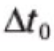. Then he will conclude that an another observer in second reference frame moving with constant speed v relative to the frame of reference, will measure time interval of.1

Now, let us study what the Twin Paradox is.

TWIN PARADOX is said to be, “A longer life, but it will not seem longer”2 .

This Paradox involves two identical clocks, one of them is kept on Earth, and the other one is brought to a journey with a speed very near to ‘c’, and then is brought back to Earth. We can replace them with two biological clocks, say twins George and Fred. It is assumed that we have made a spacecraft which can move with velocity very near to that of light in vacuum. George goes on a voyage with the spacecraft with a high velocity and Fred stays on Earth. Let us assume, George travels with v= 0.99995c.

give us,

[1- (v/c)2]1/2= 1 x 10-2= 1%.

This implies that Fred finds that, for every 100 beats of his heart, George’s heart beats only once. Similarly, for every 100 ticks of Fred’s wristwatch, George’s wristwatch ticks only once.  That is, by the time Fred’s heart beats 100 times, George’s heart beats only one time. By the time George watches Interstellar once, Fred would have watched it 100 times consecutively.

So, by the time George travels for 1 year and then returns back to Earth, Fred will have spent 100 years of his life.

Now, why is this a “Paradox”?

1. For more illustrations, see Young and Freedman, 2013.
2. For more illustrations, see Beiser, 1997.

A fundamental law of Physics is the law of Equivalence of Reference Frames. This law states that, All inertial reference frames are equivalent. Here, the reference frame of George is inertial with respect to Fred as George is not moving with acceleration with respect to Fred. So, we can see the entire problem from George’s frame of reference too.

When seeing the problem from George’s reference frame, Fred is moving with v=0.99995c. So, George should find that, Fred’s heart will beat slower than his. Now, he concludes that he will be older than Fred when they meet again.

The Paradox is that, both the twins are concluding the opposite to the other.

The first postulate of Special Theory of Relativity states that: The laws of Physics are same in all inertial reference frames. The Paradox here contradicts this postulate.

A solution to this Paradox has been made by earlier scientists. The two situations (one is George travelling with respect to Fred; other is Fred travelling with respect to George), which were once thought to be equivalent or similar, are not equivalent at all. The solution states: George is not in inertial frame at all. This is because, George has to start with acceleration, turn around during the round-trip and at last retard in order to come to rest and check who is younger. So, the Time Dilation Equation does not hold for George3.

The problem with the solution, however, is that it is observed from Fred’s reference frame. But if we see the problem from George’s reference frame, then Fred, along with the Rest of Universe, starts at first with acceleration, turn at the middle of journey and at last retards to stop. So, from George’s reference frame, Fred’s frame is non-inertial too. So, Time-Dilation

Equation should not hold for Fred’s frame too if it does not hold for George’s frame. Otherwise,

it again violates the equivalence of reference frame.

Another solution to this Paradox has been made by using the Length Contraction Equation.  We have Einstein’s this Equation as follows: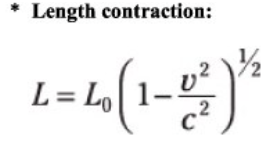The solution states that, the length of the path travels by George contracts with respect to himself. If the total length of the path of travel, measured from Earth (or as  measured by Fred) is L0, then, from George’s frame, it is (L0/100). From George’s frame, Fred is moving with V=0.99995c and the path traversed by Fred is (L0/100). So, the time required for Fred to travel, as measured by George, is L0/(100xV). However, from Fred’s frame, the length of the path is still L0, and from his frame, George is moving with V=0.99995c. So, the time required for George to travel, as measured by Fred, is (L0/V) which is 100 times higher than that measured by George.

1. For more illustrations, see Beiser, 1997.

This solution does solve the Twin Paradox however it fails to solve another Paradox. Einstein predicted that moving clock runs slower with respect to stationary one. This Paradox is not solved by the former solution.

In this present study, we will try to find out a solution which solves Twin Paradox as well as explains why moving clock runs slower than a stationary clock.

WORK:

We introduce a thought-experiment of ours, which is as follows:

Let us imagine, our brave heart Maria, is going for a time-travel. We have built a time machine, which can move with v=0.99995c. Maria starts the engine, the time-machine starts moving and reach to a velocity v=0.99995c. Thus, Maria travels across time.

Now, the assumptions of the thought-experiment are as follows:

(i)We consider there are two frames in the entire universe, for simplicity. One is the Time-Machine with Maria, and other is Rest of Universe.

(ii) Maria starts the engine and reaches to velocity v=0.99995c from v=0 in no time. During the entire journey, she travels with constant velocity v. And at the end of the journey, she instantly stops from v=0.99995c to v=0.

In a 3-dimension problem of kinematics, we often have to find out relative position, relative velocity and relative acceleration. In these cases, we have to consider the position, velocity and acceleration of the origin as standards. Then we find the relative position, relative velocity and relative acceleration of a body with respect to that of the origin. The case of the present study includes 4 dimensions. So, in this 4-d plane, we have to take all of the 4 coordinates of the origin as standards. And then, we can find relative position, relative velocity, relative acceleration and relative time of a body with respect to the origin.

How to consider the ‘time’ coordinate of the origin as a standard? It is similar to taking the other three coordinates as standards. At any instant when we are going to find the relative time of an event with respect to origin, we have to take as if the origin is in ‘Present’. Then, we can find whether the event is in ‘Past’ or in ‘Future’ with respect to the time of origin and by how much time it is leading or lagging.

Let us take an example. Suppose, we have to find the relative time of an event with respect to our time. So, our time is ‘Present’. And, we can now find the relative time of the event with respect to us. Now suppose, 100 years have passed since this measurement. Now it is year 2115. We again are going to measure the event’s or another event’s relative time with respect to our time. So, now, we have to consider this moment of 2115 as the ‘Present’.

The following figure also explains it:The above figure is when we have to find the relative time of Maria with respect to ours. Similar figure can be drawn for finding the Rest of Universe’s time with respect to Maria’s.

Now, if Maria travels with velocity v=0.99995c with respect to us, then what will happen?  Will Maria travel in time?

From the Time-Dilation Equation, we know that Maria should travel in time with respect to us. We also have our practical proof in support of it. The Neutrinos4 in the Large Hadron Collider5 are time-travelers. When they are at rest with respect to observer, their lifetime is so small that human-made machine cannot detect them. However, when they are travelling with very high speed within LHC, according to Time-Dilation Equation, their lifetime increases with respect to us. And, then we can detect them.

Similar thing happens for the Muons6. Naturally, Muons are formed high above from the sea-level, because of the collisions between cosmic rays and atmosphere. But, Muons are found in the sea-level too. This can only happen if they travel to sea level. But, Muons have decay time of about 2.2x10-6 and they travel with approximately v=0.998c. Then, from simple calculations, they can travel a maximum distance of d=vt= (0.998x3x108x2.2x10-6x10-3) km

= 0.66 km, much low.

But, as they are travelling with v=0.998c with respect to us, so according to Time-Dilation Equation, their life-time becomes: t= 2.2x10-6/ (1-0.996)1/2= 34.8x10-6.

Now, d= vt= (0.998x3x108x34.8x10-6x10-3) km = 10.4 km.

Here, with respect to the observer, the Muons are time-travelers. So, if Maria travels with v=0.99995c with respect to us, she also travels in time with respect to us.

1. Neutrino is an electrically neutral elementary particle with half-spin. Theoretically, it seems to be massless, but evidences are there to show that it has mass, no matter how small the mass can be. Neutrino is lepton, like charged electron, muon and tau. Neutrino is uncharged, so is unaffected to Electro-magnetic force. It is lepton, so is not affected by Strong-Force. It is affected only by Weak-Force and Gravity. However, weak-force is low-ranged and gravity is weak for subatomic particles. So, Neutrino typically can pass through our bodies, and is not detected. For this strange nature, it is also said “Ghost-Particle”. In LHC, it travels with very high speed and is said to travel in time.
2. LHC, world’s largest and most powerful particle collider, is undoubtedly the most complex lab ever built. It was built from 1998 to 2008. It runs from CERN, near Geneva of Switzerland, to Sasso of Italy. The aim of it is to test different proposals of Particle Physics and High-Energy Physics, especially to find the existence of Higgs-Bosons. This is the particle which is said to be the reason behind matters having ‘mass’.
3. Muon is elementary particle, with charge of -1e, like electron, and spin ½. Its mass is 105.7 MeV/c2.  It is a lepton, like electron, tau and neutrino.

Now the question is, will Maria go back in time or forth in time?

To answer this question, we have to see the problem first from our reference frame, then from Maria’s reference frame. So, now the question is, will Maria go back in time or forth in time with respect to us?

We have our time dilation equation:.Let us take an interval as 'the interval when the second hand of a clock ticks for once, that is 1 second in a particular frame of reference.'

Then, putting v=0.99995c in [1- (v/c)2]1/2give us,

[1- (v/c)2]1/2= 1 x 10-2= 1%.

In this case, Maria is in motion with respect to us. So, for every 100 ticks of the second hand of our clocks, Maria’s clock’s second hand ticks only once. So, our 100 seconds is equivalent to her 1 second. Thus, her biological clock is also slowing down and she is aging less than us.

Now, from our reference frame, Maria is travelling for the same years that we spend (here it is100 years). So, while travelling, if her time goes forth with respect to our time, then at the end of voyage she would age more than us (100+x years; if v=0.99995c then x=99 years). And, if her time goes back with respect to our time, while travelling, then at the end of voyage she would end aging less than us (100-x years).

This implies that, with respect to our time, Maria’s time is going to ‘Past’ while she travels.

Now, let us study what is seen where Maria is going, when seen from Maria’s reference frame. From the theory of equivalence of reference frame, Maria should see that the Rest of Universe is travelling in time. With respect to her time, the time of Rest of Universe should go to ‘Past’. So, every artificial and biological clock of the Rest of Universe will go to ‘Past’ with respect to Maria. Now, the question is who will end up as older.

Now, let us study the following figure.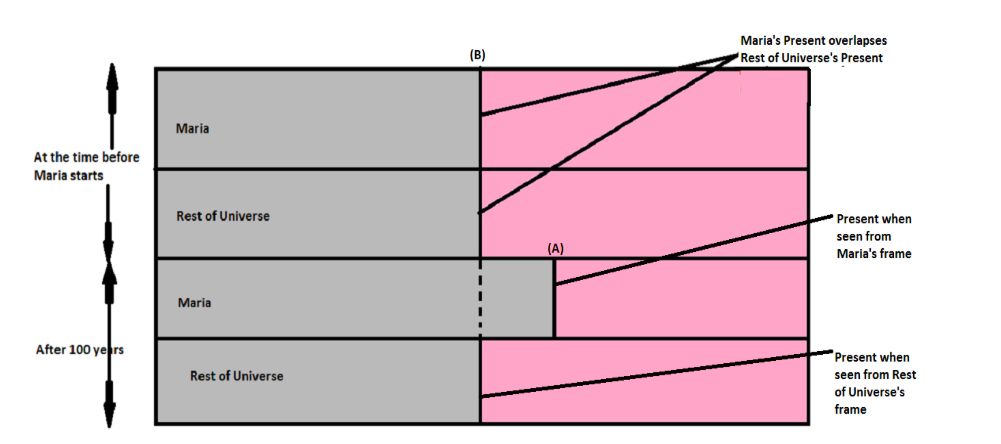It appears from the above figure that before the start of the voyage, Maria’s time and the Rest of Universe’s time overlapse. However, when the voyage starts, Rest of Universe’s time is going to ‘Past’ with respect to Maria’s time. So, Maria’s time is going to ‘Future’ with respect to Rest of Universe’s time.

If we see from Maria’s frame, her time is ‘Present’ at every instant. So, Maria will conclude that, the Rest of Universe’s ‘Present’ is her ‘Present’ and their ‘Present’s would over lapse all the time if there were no voyage of the Rest of Universe. Their ‘Presents’ overlap until Rest of Universe starts its voyage. After the moment, Rest of Universe’s time is going to ‘Past’ with respect to Maria’s time- Present. So, Rest of Universe’s time is going to ‘Past’ of itself. Studying the above figure, we can say, so, Maria is going to Rest of Universe’s ‘Future’. And, as she will conclude that she is ‘Present’ now, so, she will conclude that she will spend 1 year (as seen from her reference frame) whereas, the Rest of Universe is aging as she is going to its ‘Future’.

We can take any two Frames having relative velocity between themselves, and apply this method.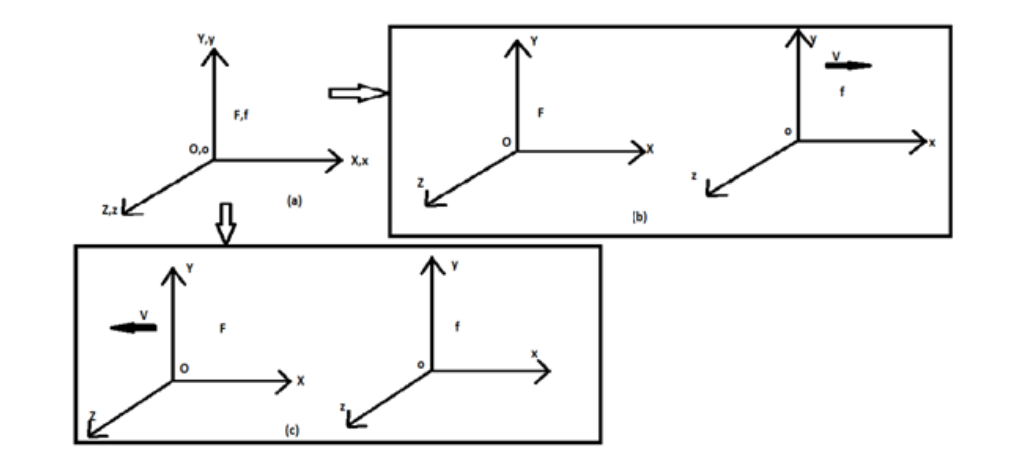In figure above, we have two Frames of references. At (a), both the Frames F and f are in the same position at time t=0.

At (b), Frame f is moving with velocity v relative to F.

At (c), Frame F is moving with velocity -v (as it is in the opposite direction to v) relative to f.

Now, each of the Frames travels in time with respect to other.

Let F concludes that f is going to f's Past throughout f's motion.

Then, f will conclude that f is going to F's Future throughout F's motion. This is equivalent to f’s conclusion of F is going to F's Past throughout F's motion.

The Observations:

If we see a body moving (i.e. the body is moving with respect to our frame of reference), we will conclude that its time is going to Past with respect to our time.

Anything, from a celestial body moving with respect to us, to the trees, houses which seem to  move when we travel by train, is going to Past with respect to our time.

It deserves mention that the proposition revealed out from the above theoretical construction may support other conclusions which are as follows:

(i) As motion is relative, so going to Past with respect to the observer, is also relative. And, thus, there is no absolute Past, Present or Future, but actually they are relative.

(ii) From our conclusion we can say that a clock moving with respect to us, is going to Past with respect to us. Einstein had said that ‘moving clock runs slower’. So, there must be a link in between our conclusion and Einstein's conclusion. Let us try to find it.

Suppose, t=0 is the initial time. We are looking for an incident to happen at t=t. Let us say, the incident is, the second-hand of a clock ticks for once. Now, the clock is moving with respect to us. So, it is going back in time every instant with respect to us. So, when we conclude, after watching a stationary watch, say our wrist watch, that one tick(or 1 second) has happened, i.e., t=t has come, the moving clock still does not reach to t=t. So, its second-hand still does not tick for once. So, the moving clock is going slow with respect to our wrist-watch. So, the moving clock is running slower.

(iii) Our conclusion supports the equivalent nature of Reference Frames.

(iv) An another Paradox can be solved by our method. It is the Rocket Warfare Paradox7. Consider, two identical rockets A and B of respective length l0 in their respective frames. When seen from B’s frame, length of A is l0/k, where k=1/(1-v2/c2). Similar is for A’s frame. Consider the situation when, as seen from B, a missile is fired to A from B when tip of B coincides with tail of B. In this case, the missile misses the target A.The above figure is viewed from B. Now, when seen from A,the missile  should blast A up.

Solution to this paradox is Simultaneity. We used a concept of Simultaneity when we supposed that the missile was launched when tip of B met tail of A. But these two events of launching and the meeting of tip of B to tail of A are simultaneous from B’s frame. But they were not simultaneous from A’s frame.

Now, let us try to solve it using the conclusive observation of present study.

1. For more illustrations, see Mani and Mehta, 2000.

If we see from A’s frame, B’s time is going to Past with respect to A’s time. A’s Present and B’s Present would overlap if there were no relative motion between them, that is, no time difference would occur in between their respective measurements. So, from A’s frame, B’s Present is A’s Present and B is going to Past with respect to B’s Present.

And, if we see from B’s frame, B’s Present is what is seen from B’s frame, where the launching of missile occurs when tip of B coincides with tail of A.

So, from A’s frame, the launching of missile from B must occur before the tip of B coincides with tail of A. Thus, these two events are not simultaneous from A.

We can find how the time of one reference frame increases while of second frame decreases, that is one’s time is going to Past and other’s is going to Future, with the help of Lorentz Transform.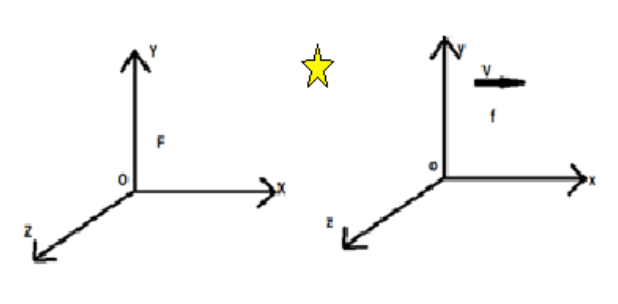Suppose, there are frame-f and frame-F. An explosion occurs as shown. Now, our concern is how the time of explosion changes with respect to f and F.

At any instant, we can imagine a frame f*, in between f and F, as shown: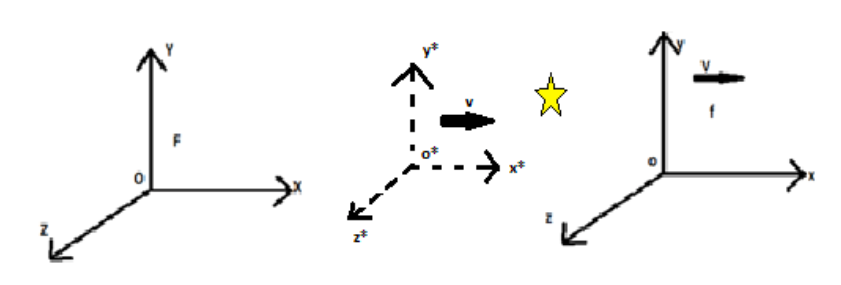Here, mod(V)> mod(v).

So, from frame f*, velocity of frame F is –v.

And, from frame f*, velocity of frame f is (V-v).

From Lorentz Transform, we know, t=k(t0 – xv/c2), where k= (1 – v2/c2)-1/2.

So, from frame f*, for frame f:

tf =  k[t0 – x(V-v)/c2]

And, from frame f*, for frame F:

tF =k(t0 + xv/c2)

And k>= 1.

So, tf< t0< tF.

So, from frame f*, frame F’s time is increasing, however, frame f’s time is decreasing.

So, with respect to a specific time t0, f’s time is going to Past, where as F’s time is going to Future.

Let us study the following figure:In this case, x is negative. So,

tF= k(t0 - xv/c2)

And tf = k[t0 + x(V-v)/c2].

So, here tF< t0< tf.

So, with respect to a specific time t0, F’s time is going to Past, where as f’s time is going to Future.

It is amazing to see in former case, f’s time was going to Past, and F’s to Future whereas in second case the vice versa happens. But it is mostly like to phenomena when we run towards the finish line in a race. As we move, the finish line comes closer to us. But as we touch and cross it, and move further ahead, the finish line goes further away. Similarly, as we live, we go closer to event of man landing on Mars. But as we will land, and live more, we will be going further away from the event.

Thus, we see how the time of one frame goes to Past with respect to an event, where as the time of other frame goes to Future with respect to the event.

Now let us recall the paradox. George is moving with v=0.99995c by a space craft while Fred remains on earth. As both of them see the other moving, so both may to conclude that, after they meet again, the other one will be younger.

Now, let us use our conclusion of the thought-experiment to this Paradox. We can assume George with the space-craft as one frame and Rest of Universe with Fred as the other frame. Now, we can simply imagine George’s frame in place of Maria’s.

George travels with velocity v=0.99995c, as seen by Fred, i.e. George is in motion with respect to Fred. So, Fred will conclude that George has gone to Past, as we conclude for Maria. Using the Time-Dilation equation, or by using the conclusion of the present study, he will conclude that George’s time is lagging with respect to his time. So, George will end up as younger.

George will see everything besides himself is in motion, so will conclude, "Everything besides himself is going back in time with respect to him. So, he himself is going forth of the Rest of Universe’s time. So, he is going to ‘Future’ of Fred. So, at end of voyage he will see ‘Future’ Fred – that is, older Fred.

REFERENCES:

Beiser, A. (1997): Concepts of Modern Physics, New Delhi: Tata McGraw-Hill Publication.

Mani, H. S. and Mehta, G. K. (2000): Introduction to Modern Physics, New Delhi: East West Press.

Young, H. D. and Freedman, R. (2013): University Physics, 12th Edition, New Delhi: Pearson Publication..